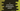# 3 different C programs to print a hollow parallelogram star pattern## C program to print a hollow parallelogram pattern:

In this post, we will learn how to print a hollow parallelogram pattern in C. The program will take the total number of rows and columns as inputs from the user and print the hollow parallelogram using star or any other characters. For example, the below is a hollow parallelogram of 6 rows and 5 columns:

``````     * * * * *
*       *
*       *
*       *
*       *
* * * * * ``````

### Algoithm to print a hollow parallelogram:

Before we start writing the algorithm, let me replace all blank spaces with #:

``````####* * * *
###* # # *
##* # # *
#* # # *
* * * * ``````
• This is a parallelogram of 5 rows and 4 columns.
• For the first row, it printed 4 blank spaces and then it printed 4 stars.
• For the second row, it printed 3 blank spaces and then 1 star, 2 spaces and 1 star.
• For the third row, 2 blank spaces, 1 star, 2 spaces and 1 star etc.

So, we have to use three loops:

• The first loop will point to each row of the parallelogram.

• The second loop will run in the first loop, we will use it to print the starting spaces.
• The third loop will run to print the content of the parallelogram.
• Ask the user to enter the total number of rows and columns. Read these values and assign the values to different variables.
• Let say, the first loop runs from i = 1 to i = rows. This is the outer loop.

• The first inner loop will run from j = 1 to j = rows - i to print the starting spaces.
• The second inner loop will run from k = 1 to k = columns. This loop will print star if i = 1 or i = rows or k = 1 or k = columns. Else, it will print a blank space.
• At the end of each iteration of the outer loop, print a new line character to move the control to the next line.

### Example 1: C program to print a hollow parallelogram by using for loops:

This example uses for loops to print the hollow parallelogram. It will use the above algorithm to print the pattern.

``````#include <stdio.h>

int main()
{
int row, col;

printf("Enter the total number of rows: ");
scanf("%d", &row);

printf("Enter the total number of columns: ");
scanf("%d", &col);

for (int i = 1; i <= row; i++)
{
for (int j = 1; j <= row - i; j++)
{
printf(" ");
}

for (int k = 1; k <= col; k++)
{
if (i == 1 || i == row || k == 1 || k == col)
{
printf("* ");
}
else
{
printf("  ");
}
}
printf("\n");
}
return 0;
}``````

If you run this program, it will print output as below:

``````Enter the total number of rows: 7
Enter the total number of columns: 5
* * * * *
*       *
*       *
*       *
*       *
*       *
* * * * * ``````

### Example 2: C program to print a hollow parallelogram by using while loops:

Let’s use while loops to print the hollow parallelogram. It will be almost similar to the above program.

``````#include <stdio.h>

int main()
{
int i = 1, j, k, row, col;

printf("Enter the total number of rows: ");
scanf("%d", &row);

printf("Enter the total number of columns: ");
scanf("%d", &col);

while (i <= row)
{
j = 1, k = 1;

while (j <= row - i)
{
printf(" ");
j++;
}

while (k <= col)
{
if (i == 1 || i == row || k == 1 || k == col)
{
printf("* ");
}
else
{
printf("  ");
}
k++;
}
printf("\n");
i++;
}
return 0;
}``````

It will print similar results.

``````Enter the total number of rows: 10
Enter the total number of columns: 5
* * * * *
*       *
*       *
*       *
*       *
*       *
*       *
*       *
*       *
* * * * * ``````

### Example 3: C program to print a hollow parallelogram with user input character:

Let’s take the character also as input. We will add one more character variable to hold the character and we will print the value of this variable instead of stars:

``````#include <stdio.h>

int main()
{
int i = 1, j, k, row, col;
char c;

printf("Enter the total number of rows: ");
scanf("%d", &row);

printf("Enter the total number of columns: ");
scanf("%d", &col);

getchar();
printf("Enter the character to print the pattern: ");
c = getchar();

while (i <= row)
{
j = 1, k = 1;

while (j <= row - i)
{
printf(" ");
j++;
}

while (k <= col)
{
if (i == 1 || i == row || k == 1 || k == col)
{
printf("%c ", c);
}
else
{
printf("  ");
}
k++;
}
printf("\n");
i++;
}
return 0;
}``````

Output:

``````Enter the total number of rows: 5
Enter the total number of columns: 3
Enter the character to print the pattern: *
* * *
*   *
*   *
*   *
* * *

Enter the total number of rows: 5
Enter the total number of columns: 3
Enter the character to print the pattern: ^
^ ^ ^
^   ^
^   ^
^   ^
^ ^ ^ ``````